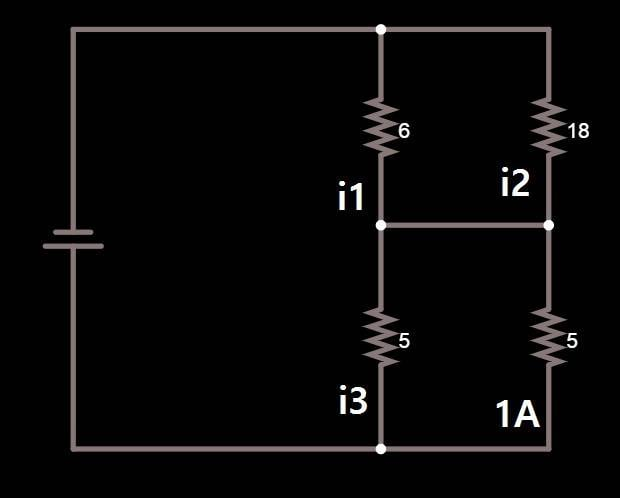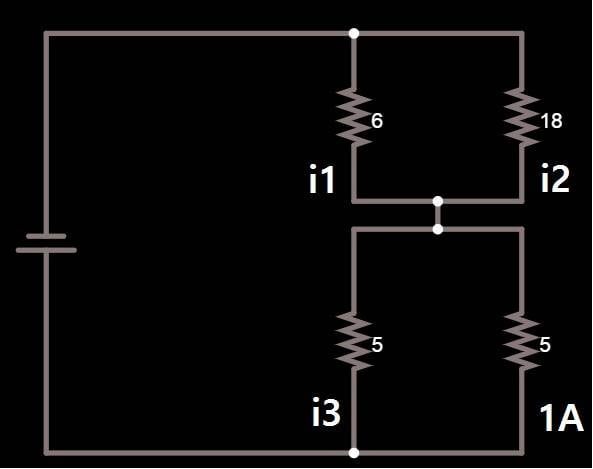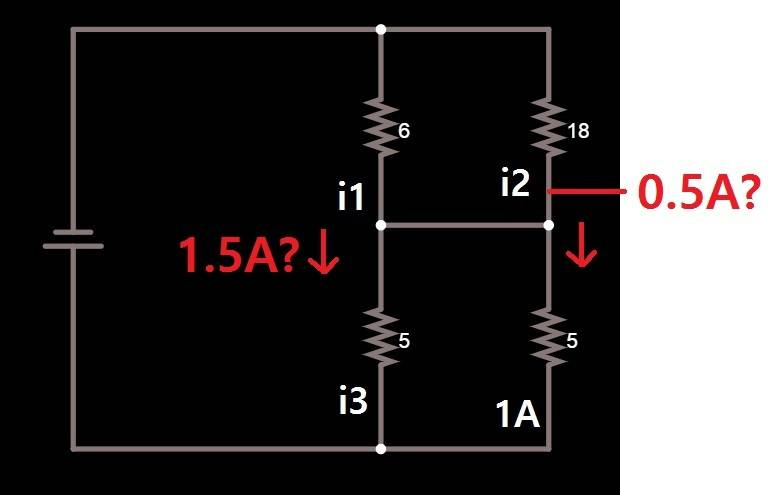# Simplifying a series / parallel circuit and calculating unknown values

jimmystevens123
Homework Statement:
I'm struggling with interpreting the circuit correctly, and checking my work afterwards yields confusing results.
Relevant Equations:
Kirchhoff's Current Law
Ohms LawGiven the circuit above, I have to solve for the labelled currents, find V total and R total accordingly. 1A is flowing through the 5Ω resistor as shown. Assuming electron flow (negative terminal to positive) for circuit.

The connector in the middle was somewhat confusing. Without it, this would be much simpler. At any rate, I redrew the circuit (perhaps incorrectly) as follows:If I have a 1A going through one identical parallel resistor, I must have 1A going through the other.

Adding the equivalent parallel resistor values, I get 4.5 + 2.5 = 7Ω.

2A across 2.5Ω = 5V dropped.
2A across 4.5Ω = 9V dropped.

R total = 7Ω
I total= 2A
V total = 14V

Using Kirchoffs Current Law - (i2 = It * R1 / R1+R2) 2A * 6/6+18 = 0.5A?
i1 = 2A * 18/6+18 = 1.5A?

I feel like the redraw was a mistake, because without that junction, the values don't make any sense in the original circuit.My question is, how do I interpret this circuit correctly? How do I know if any current is going to flow through the middle connector, and if so, how much? It's not intuitive to me at all. Apologies for the length.

Homework Helper
Gold Member
The thing to think about the tie connection in the middle - the voltage is the same at that point.

If it is an ideal conductor (0 ohms) then regardless of the amount of current through it, there is zero voltage drop. This makes the two bottom resistors in parallel, and the two top ones in parallel.

The way you redrew it is electrically the same as the original drawing.

Your calculations look correct - I would add some parentheses to make it a little more clear.

So if 1.5 A flows through R1, and 0.5 A flows through R2, and you have the currents in the bottom two resistors, how much flows through the tie connector?

Last edited: